# 5.2 Thermophysical models

Thermophysical models are used to describe cases where the thermal energy, compressibility or mass transfer is important.

OpenFOAM allows thermophysical properties to be constant, or functions of temperature, pressure and composition. Thermal energy can be described in form of enthalpy or internal energy. The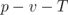relation can be described with various equations of state or as isobaric system.

The thermophysicalProperties dictionary is read by any solver that uses the thermophysical model library. A thermophysical model is constructed in OpenFOAM as a pressure-temperature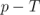system from which other properties are computed. There is one compulsory dictionary entry called thermoType which specifies the complete thermophysical model that is used in the simulation. The thermophysical modelling starts with a layer that defines the basic equation of state and then adds further layers for the thermodynamic, transport and mixture modelling, as listed in Table 5.1.

 Equation of State — equationOfState icoPolynomial Incompressible polynomial equation of state, e.g. for liquids perfectGas Perfect gas equation of state Basic thermophysical properties — thermo eConstThermo Constant specific heat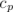model with evaluation of internal energyand entropyhConstThermo Constant specific heat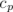model with evaluation of enthalpy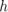and entropyhPolynomialThermo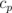evaluated by a function with coefficients from polynomials, from which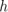,are evaluated janafThermo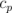evaluated by a function with coefficients from JANAF thermodynamic tables, from which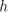,are evaluated Derived thermophysical properties — specieThermo specieThermo Thermophysical properties of species, derived from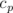,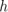and/orTransport properties — transport constTransport Constant transport properties polynomialTransport Polynomial based temperature-dependent transport properties sutherlandTransport Sutherland’s formula for temperature-dependent transport properties Mixture properties — mixture pureMixture General thermophysical model calculation for passive gas mixtures homogeneousMixture Combustion mixture based on normalised fuel mass fractioninhomogeneousMixture Combustion mixture based onand total fuel mass fraction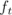veryInhomogeneousMixture Combustion mixture based on,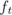and unburnt fuel mass fraction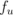dieselMixture Combustion mixture based on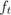and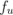basicMultiComponentMixture Basic mixture based on multiple components multiComponentMixture Derived mixture based on multiple components reactingMixture Combustion mixture using thermodynamics and reaction schemes egrMixture Exhaust gas recirculation mixture Thermophysical model — thermoModel hePsiThermo General thermophysical model calculation based on enthalpy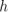or internal energy, and compressibility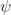heRhoThermo General thermophysical model calculation based on enthalpy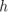or internal energy, and density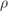hePsiMixtureThermo Calculates enthalpy for combustion mixture based on enthalpy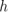or internal energy, and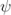heRhoMixtureThermo Calculates enthalpy for combustion mixture based on enthalpy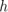or internal energy, and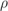heheuMixtureThermo Calculates enthalpy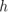or internal energyfor unburnt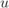gas and combustion mixture Table 5.1: Layers of thermophysical modelling.

Various combinations are available as ‘packages’, specified using, e.g.

17
18thermoType
19{
20    type            heRhoThermo;
21    mixture         pureMixture;
22    transport       const;
23    thermo          hConst;
24    equationOfState perfectGas;
25    specie          specie;
26    energy          sensibleEnthalpy;
27}
28
29mixture
30{
31    specie
32    {
33        molWeight       28.96;
34    }
35    thermodynamics
36    {
37        Cp              1004.4;
38        Hf              0;
39    }
40    transport
41    {
42        mu              1.831e-05;
43        Pr              0.705;
44    }
45}
46
47
48// ************************************************************************* //

Only certain combinations are predefined. One method to identify the possible combinations from Table 5.1 is to use a nonexistent setting for one of the entries, e.g.banana and execute the solver. OpenFOAM will issue an error message and list all possible combinations to the terminal.

### 5.2.1 Thermophysical property data

The basic thermophysical properties are specified for each species from input data. Data entries must contain the name of the specie as the keyword, e.g. O2, H2O, mixture, followed by sub-dictionaries of coefficients, including:

specie
containing i.e. number of moles, nMoles, of the specie, and molecular weight, molWeight in units of g/mol;
thermo
containing coefficients for the chosen thermodynamic model (see below);
transport
containing coefficients for the chosen transport model (see below).

The thermodynamic coefficients are ostensibly concerned with evaluating the specific heat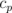from which other properties are derived. The current thermo models are described as follows:

hConstThermo
assumes a constantand a heat of fusion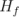which is simply specified by a two values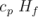, given by keywords Cp and Hf.
eConstThermo
assumes a constant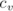and a heat of fusion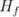which is simply specified by a two values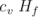, given by keywords Cv and Hf.
janafThermo
calculates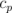as a function of temperature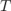from a set of coefficients taken from JANAF tables of thermodynamics. The ordered list of coefficients is given in Table 5.2. The function is valid between a lower and upper limit in temperature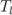and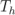respectively. Two sets of coefficients are specified, the first set for temperatures above a common temperature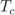(and below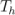, the second for temperatures below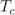(and above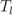). The function relating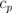to temperature is: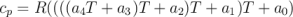(5.1)

In addition, there are constants of integration,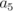and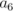, both at high and low temperature, used to evaluating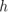andrespectively.

hPolynomialThermo
calculates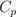as a function of temperature by a polynomial of any order. The following case provides an example of its use: \$FOAM_TUTORIALS/lagrangian/porousExplicitSourceReactingParcelFoam/filter

 Description Entry Keyword Lower temperature limit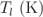Tlow Upper temperature limit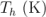Thigh Common temperature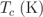Tcommon High temperature coefficients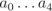highCpCoeffs (a0 a1 a2 a3 a4... High temperature enthalpy offset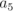a5... High temperature entropy offset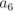a6) Low temperature coefficients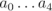lowCpCoeffs (a0 a1 a2 a3 a4... Low temperature enthalpy offset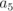a5... Low temperature entropy offset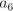a6)

Table 5.2: JANAF thermodynamics coefficients.

The transport coefficients are used to to evaluate dynamic viscosity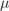, thermal conductivity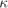and laminar thermal conductivity (for enthalpy equation)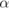. The current transport models are described as follows:

constTransport
assumes a constant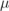and Prandtl number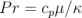which is simply specified by a two keywords, mu and Pr, respectively.
sutherlandTransport
calculates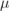as a function of temperature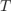from a Sutherland coefficient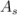and Sutherland temperature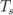, specified by keywords As and Ts;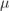is calculated according to: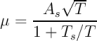(5.2)

polynomialTransport
calculates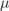and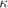as a function of temperature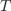from a polynomial of any order.

The following is an example entry for a specie named fuel modelled using sutherlandTransport and janafThermo:

fuel
{
specie
{
nMoles       1;
molWeight    16.0428;
}
thermodynamics
{
Tlow         200;
Thigh        6000;
Tcommon      1000;
highCpCoeffs (1.63543 0.0100844 -3.36924e-06 5.34973e-10
-3.15528e-14 -10005.6 9.9937);
lowCpCoeffs  (5.14988 -0.013671 4.91801e-05 -4.84744e-08
1.66694e-11 -10246.6 -4.64132);
}
transport
{
As           1.67212e-06;
Ts           170.672;
}
}
The following is an example entry for a specie named air modelled using constTransport and hConstThermo:

air
{
specie
{
nMoles          1;
molWeight       28.96;
}
thermodynamics
{
Cp              1004.5;
Hf              2.544e+06;
}
transport
{
mu              1.8e-05;
Pr              0.7;
}
}